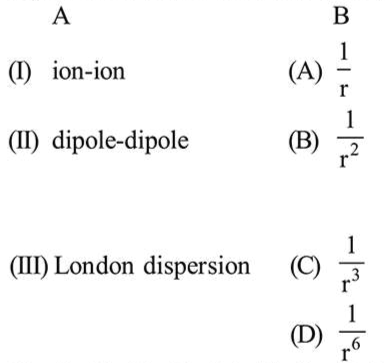# Match the type of interaction in column A with the distance dependence of their interaction energy in column B :

Question:

Match the type of interaction in column A with the distance dependence of their interaction energy in column B :1. (I)-(B), (II)-(D), (III)-(C)

2. (I)-(A),(II)-(B), (III)-(D)

3. (I)-(A), (II)-(B), (III)-(C)

4. (I)-(A), (II)-(C), (III)-(D)

Correct Option: , 4

Solution:

(I) Ion-ion interaction energy $\propto\left(\frac{1}{r}\right)$.

(II) Dipole-dipole interaction energy $\propto\left(\frac{1}{r^{3}}\right)$.

(III) London dispersion $\propto\left(\frac{1}{r^{6}}\right)$.